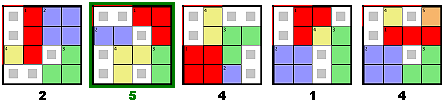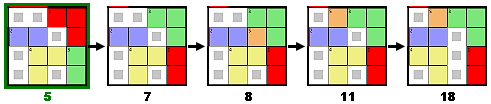# How the PuzzleBeast Puzzles are Created

All the puzzles on PuzzleBeast are created by computer program. These puzzle-generating programs are written in the Java programming language, and are referred to collectively as "beasts" after the first such program I wrote, SwampBeast.

In its simplest form the PuzzleBeast process uses two steps to create a puzzle. First, a large number of random puzzles are generated. Each puzzle is solved by the computer program and given a score that indicates how difficult the puzzle is to solve. In the example below, five randomly generated sliding block puzzles are shown. The number below each puzzle represents the number of moves in the shortest solution and is the score for the puzzle. The highest scoring puzzle is marked in green.

GenerationIn the second step, the highest scoring puzzle from the generation step is selected and randomly mutated for a number of cycles. In each cycle the mutated version of the puzzle is compared to the original, and if the new version scores higher it is kept and the original is discarded. The illustration below shows the puzzle from above going through four mutations, resulting in a puzzle with solution length of eighteen moves.

MutationThe puzzles on PuzzleBeast are typically the result of a large number of cycles (sometimes greater than 100,000 steps each of generation and mutation).

In the example above the puzzles are scored only according to the length of the shortest solution, but in practice scoring algorithms are usually more complicated and include a number of parameters chosen to make the puzzles interesting to solve.

## Mutation Examples

Here are the five puzzles from the mutation illustration above.

Goal: Move the red block to the upper left corner.

## Applet Info

• To move a block, grab it and drag.
• Undo a move - press U or click the undo arrow in the lower right.
• Redo a move - press R or click the redo arrow in the lower right.
• Next puzzle or previous puzzle - Click on the white arrows at the upper left and right corners.